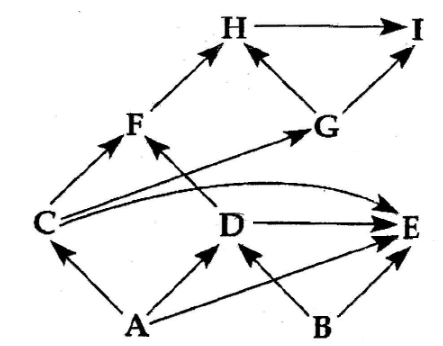# Problem: Refer to Figure below, the diagram of a food web, for the following question. (Arrows represent energy flow and letters represent species). Which species in the above figure might be described as an omnivore?a. Db. Bc. Ed. Fe. I

🤓 Based on our data, we think this question is relevant for Professor Owens' class at UCSD.

###### Problem Details

Refer to Figure below, the diagram of a food web, for the following question. (Arrows represent energy flow and letters represent species).

Which species in the above figure might be described as an omnivore?

a. D

b. B

c. E

d. F

e. IWhat scientific concept do you need to know in order to solve this problem?

Our tutors have indicated that to solve this problem you will need to apply the Community Structure concept. You can view video lessons to learn Community Structure. Or if you need more Community Structure practice, you can also practice Community Structure practice problems.

What is the difficulty of this problem?

Our tutors rated the difficulty ofRefer to Figure below, the diagram of a food web, for the fo...as high difficulty.

How long does this problem take to solve?

Our expert Biology tutor, Kaitlyn took 8 minutes and 52 seconds to solve this problem. You can follow their steps in the video explanation above.

What professor is this problem relevant for?

Based on our data, we think this problem is relevant for Professor Owens' class at UCSD.